# On Several Matrix Kantorovich-Type Inequalities

## Abstract

We present several matrix Kantorovich-type inequalities, which improve the results obtained in Liu and Neudecker (1996). Elementary methods suffice to prove the inequalities.

## 1. Introduction

Letbe a positive (semi-)definite Hermite matrix with eigenvalues contained in the intervalwhereLetbematrix, and letdenotes the column space ofA well-know matrix version of Kantorovich inequality asserts that (see)(1.1)

forandwheredenotes the conjugate transpose of the matrix.

Letbe an-by-matrix; the Moore-Penrose inverseofis defined as the unique-by-matrix satisfying all of the following four criteria (see, e.g., ):(1.2)

It is not difficult to see that if, then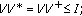we can get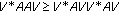; thus,forIn paper , from(which is equivalent to (13) in ), Liu and Neudecker presented the following so-called Kantorovich-type inequality:(1.3)

forandand the following inequality: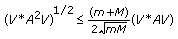(1.4)

for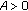and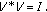Furthermore, in the same way, they obtained three more general versions.(1.5)(1.6)(1.7)

forandIn the next section, we shall present several similar matrix Kantorovich-type inequalities, which improve some results above.

## 2. New Matrix Kantorovich-Type Inequalities

We first introduce two lemmas.

Lemma 2.1.forandProof.

It is easy to see that ifthenthus, we have(2.1)

forIn , Dragomir defines a transformfor this transform, we have the following lemma.

Lemma 2.2.

Letthen(2.2)

thus(2.3)

forandProof.(2.4)

From Lemma 2.2, we can easily get the inequality (1.4).

Corollary 3.2.forandProof.

From, we have(2.5)

then(2.6)

The proof is completed.

Theorem 2.4.forandProof.(2.7)

From Lemmas 2.1 and 2.2, we have(2.8)

The proof of Theorem 2.4 is completed.

Remark 2.5.

It is not difficult to see that ifthen we conclude that Theorem 2.4 gives an improvement of the Kantorovich inequality (1.3).

Furthermore, in similar way we got Theorem 2.4, and we obtain three more general versions, which also improve the inequalities (1.5), (1.6), (1.7), respectively.

Theorem 2.6.(2.9)(2.10)(2.11)

forandwhereProof.

In fact, they are equivalent by noting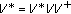and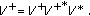For (2.9), pre- and postmultiplying by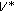andrespectively, we get the inequality (2.10); similarly, for (2.10), pre- and postmultiplying byrespectively, we get the inequality (2.11). So, we only prove the inequality (2.9).

Similarly, with Lemma 2.2, we have(2.12)

Remark.

From the proof, it is easy to see thatso, we conclude that the inequality (2.9) gives an improvement of the inequality (1.5), meanwhile, the inequalities (2.10) and (2.11) improve the inequalities (1.6) and (1.7), respectively.

## References

1. Mond B, Pečarić JE: A matrix version of the Ky Fan generalization of the Kantorovich inequality. Linear and Multilinear Algebra 1994, 36(3):217–221. 10.1080/03081089408818291

2. Wang SG, Shao J: Constrained Kantorovich inequalities and relative efficiency of least squares. Journal of Multivariate Analysis 1992, 42(2):284–298. 10.1016/0047-259X(92)90048-K

3. Chen L, Zeng X-M: Rate of convergence of a new type kantorovich variant of bleimann-butzer-hahn operators. Journal of Inequalities and Applications 2009, 2009:-10.

4. Golub GH, Van Loan CF: Matrix Computation. Johns Hopkins University, Baltimore, Md, USA; 1983.

5. Liu S, Neudecker H: Several matrix Kantorovich-type inequalities. Journal of Mathematical Analysis and Applications 1996, 197(1):23–26. 10.1006/jmaa.1996.0003

6. Marshall AW, Olkin I: Matrix versions of the Cauchy and Kantorovich inequalities. Aequationes Mathematicae 1990, 40(1):89–93. 10.1007/BF02112284

7. Dragomir SS: New inequalities of the Kantorovich type for bounded linear operators in Hilbert spaces. Linear Algebra and Its Applications 2008, 428(11–12):2750–2760. 10.1016/j.laa.2007.12.025

## Acknowledgments

The authors would like to thank the anonymous referees for their valuable comments which have been implemented in this revised version. This work is supported by Natural Science Foundation of China no.10961010, Natural Science Foundation of Jiangxi, China no 2007GZS1760, and scientific and technological project of Jiangxi education office, China no GJJ08432.

## Author information

Authors

### Corresponding author

Correspondence to Zhibing Liu.

## Rights and permissions

Reprints and Permissions

Liu, Z., Lu, L. & Wang, K. On Several Matrix Kantorovich-Type Inequalities. J Inequal Appl 2010, 571629 (2010). https://doi.org/10.1155/2010/571629

• Revised:

• Accepted:

• Published:

• DOI: https://doi.org/10.1155/2010/571629

### Keywords

• General Version
• Matrix Version
• Kantorovich Inequality